Parents and Teachers: Support Ducksters by following us onor.

Kids Math

Polygons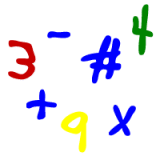A polygon is a flat figure that is made up of straight lines and is enclosed.

A few notes on the definition of a polygon that hopefully will help you remember:
• Flat - this means it's a plane figure or two-dimensional
• Straight lines - these are called segments in geometry
• Enclosed - all the lines fit end-to-end and form a figure with no openings.
More on what enclosed means:

The following figures are not enclosed and are not polygons: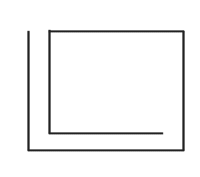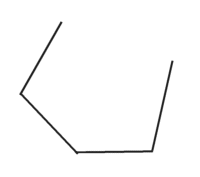The following figures are enclosed and are polygons: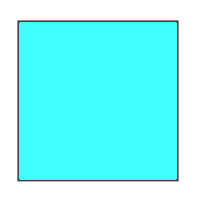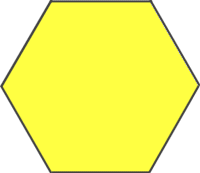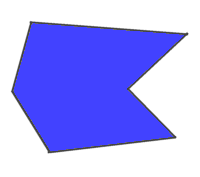Types of Polygons

There are a lot of types of polygons. Some you've probably heard of before like squares, triangles, and rectangles. We'll learn more about these and others. Polygons are named for the number of sides they have. Here is a list of polygon names depending on the number of sides they have, starting with three and ending with ten.
• 3 sides - Triangle
• 5 sides - Pentagon
• 6 sides - Hexagon
• 7 sides - Heptagon
• 8 sides - Octagon
• 9 sides - Nonagon
• 10 sides - Decagon
There are, of course, polygons with many more names and sides. When the number of sides gets really high, mathematicians sometimes use the number of sides "n" and call it an n-gon. For example if a polygon has 41 sides, it would be called a 41-gon.

Convex or Concave Polygons

A polygon is either convex or concave. It's convex if any line drawn through it intersects only two other lines. If any line drawn through the polygon can hit more than two other lines, then it's concave.

Examples:Concave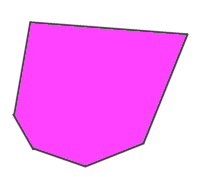Convex

In a convex polygon, every angle is less than 180 degrees. In a concave there is at least one angle greater than 180 degrees.

Simple and Complex Polygons

In a simple polygon the lines don't intersect. In a complex polygon the lines intersect.

Examples: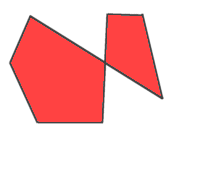ComplexSimple

Regular Polygons

A regular polygon has lines that are all the same length and it also has all the same angles.

Examples:

Regular: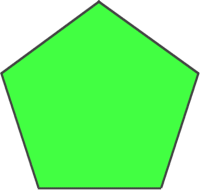Not regular:More Geometry Subjects

Circle
Polygons
Triangles
Pythagorean Theorem
Perimeter
Slope
Surface Area
Volume of a Box or Cube
Volume and Surface Area of a Sphere
Volume and Surface Area of a Cylinder
Volume and Surface Area of a Cone
Angles glossary
Figures and Shapes glossary

Back to Kids Math

Back to Kids StudyFollow us onor